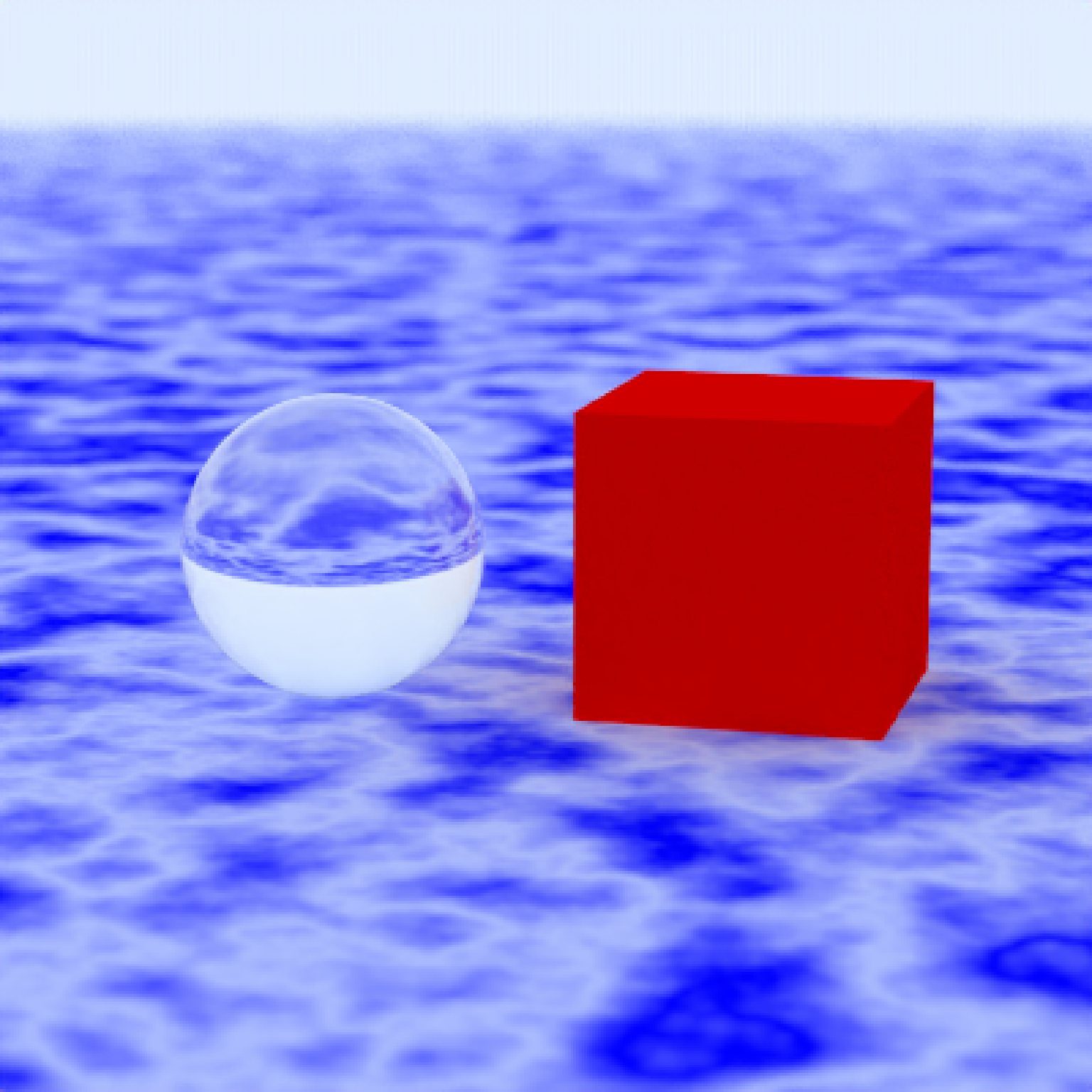Generates a large sphere that can be used as the ground for a scene.

generate_ground(depth = -1, spheresize = 1000,
material = lambertian(color = "#ccff00"))

## Arguments

depth Default -1. Depth of the surface. Default 1000. Radius of the sphere representing the surface. Default lambertian with color= "#ccff00".The material, called from one of the material functions lambertian, metal, or dielectric. Default #ccff00. The color of the sphere. Can be either a hexadecimal code, or a numeric rgb vector listing three intensities between 0 and 1.

## Value

Single row of a tibble describing the ground.

## Examples

#Generate the ground and add some objects
scene = generate_ground(depth=-0.5,material = lambertian(noise=1,noisephase=10)) %>%
add_object(cube(z=0.7,material=metal(color="red",fuzz=0.025),angle=c(0,-15,0))) %>%
add_object(sphere(z=-0.7,radius=0.5,material=dielectric(color="green")))render_scene(scene)